top of page
Search

# Numpy Part 1

## Introduction

1. NumPy stands for Numerical Python

2. NumPy is an important library for scientific computing in Python

3. It provides a high-performance multidimensional array object, and tools for working with these arrays

4. Using this NumPy , we can perform mathematical and logical operation on arrays

## Arrays

1. An array is a data structure that contains a group of elements

2. All the elements in the array are of same data types

3. All the elements in the array have an index value

4. Index value starts from 0

5. If we go from left to right then the index value will be a postive value

6. If we go from right to left then the index value will be a negative value## Installation

1. Python does not come with NumPy module . So we need to install it using pip (Python package installer)

2. Open idle or any other tools where you are coding and type the below code and run it

`pip install numpy`

## Ndarray Object

1. NumPy Provides with an N-dimensional array object called ndarray

2. ndarray describes the collection of items of the same type

3. Items in the collection can be accessed using a zero based index as it is an array

4. ndarray object consists of contiguous one dimensional segment of computer memory

5. The memory block holds the element in a row major order or column major order

6. Here N means the no of dimension it can be 1 ,2 or upto N dimension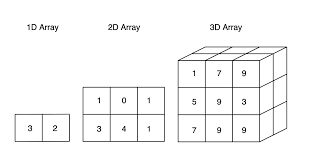### N -Dimension

• 1D array can be called as a vector ( imagine as a single row or column [only one axis])

• 2D array can be called as matrix ( imagine as combination of rows and columns example a table [two axis])

• more than 3d can be called as tensors ( imagine as a Stacking of matrixes )

## Data Type Objects

1. Each element in an ndarray is an object if data-type object called dtype

2. A data type object describes a fixed block of memory

## Array Creation

1. The default ndarray is created using an array() function in NumPy

`numpy.array()`
• It creates an ndarray object from any object like list,tuple etc,or from any mothod that returns an array

`numpy.array(object,dtype = None , ndmin = 0) `
• the above function takes the following parameters to create an ndarray object

1. object ------> Any object following the array interface ( you can use list ,tuple etc.)

2. dtype ------> Data Type of array elements (Default will be float data type)

3. ndim ------> Minimum dimension of resultant array ( N value)

• Before using this functions import numpy module by using import keyword

`import numpy as np`

** "as" is used to create an alias (temproary name)

### 1) object

Example 1Example 2### 2) dtype (Data Types)

1. NumPy supports a wider variety of numerical data types than Python

2. dtype parameter can be used inside numpy() to change the datatype of the object

Data Types Description

1. bool_ True or False

2. int8 Byte(-128 to127)

3. int16 Byte(-32768 to 32767)

4. int32 Byte(-2147483648 to 2147483648)

5. int64 Byte(-9223372036854775808 to 9223377......)

6. uint8 unsigned integer (0 to 255)

7. uint16 unsigned integer (0 to 65535)

8. uint32 unsigned integer (0 to 4294967295)

9. uint64 unsigned integer (0 to 1844674407......)

10. float16 Decimal values

11. float32 Decimal values

12. float64 Decimal values

13. complex64 Complex number

14. complex128 Complex number

• Dtype also support python data types

• Each NumPy data types has a character code which we can use in dtype parameters

Character Code Data Types

• "b" boolean

Example 3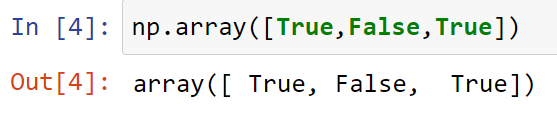• "i" signed integer

Example 5• "f" floating numbers

Example 5• "S" strings

Example 6• "O" python objects

Example 7• in dtype we can use other numpy dtype objects using numpy module

Example 8### 3) ndim

1. ndim parameter is used to provide the rank (dimension) of the array (ex:- 1,2,3,4...n dimension)

Example 9

• Scalar value no dimension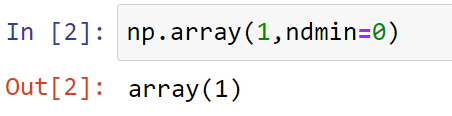Example 10

• Vector value 1 dimensionExample 11

• Matrix value 2 dimension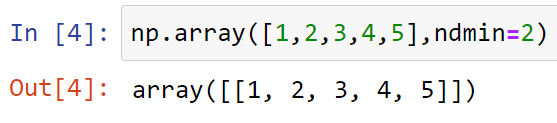Example 12

• Tensors more than or equal to 3 dimension## Array Attributes

• Array object have lots of attributes (attribute is a specification that defines a property of an object)

### shape

• This attributes returns shape of the array

• shape of the array will be in a tuple format consisting of array dimensions

Example 13

• 1 dimension array will return a tuple (n,) where "n" is the number of elements in the arrayExample 14

• 2 dimension array will return a tuple (n,m) where "n" is the no of rows and "m" is the no of columnsExample 15

• 3 dimension array will return a tuple (q,n,m) where "q" is the depth , "n" is the no of rows and "m" is the no of columns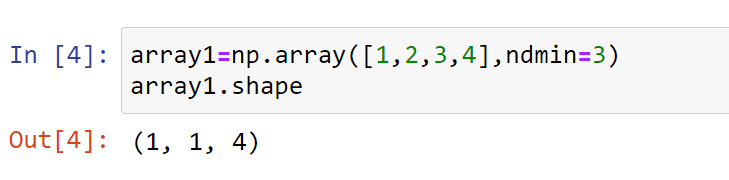### ndim

• This array attributes returns the rank of the array (dimensions)

Example 16### dtype

• This attribute returns the data type of the array

Example 17### size

• This attribute returns the no of elements in the array

Example 18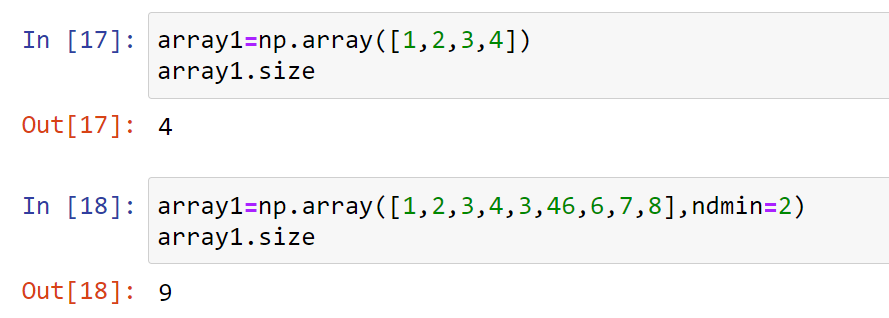## Different Functions To Create An Array

• Array object can be created by using default array() functions

• But there are lots of diiferent functions by using which we can create an array object let's see ..

### zeros((shape))

• Returns an array where all the elements are zeros

• Use a tuple inside the zeros function ,which defines the shape of an array

Example 19*** default dtype will be float that's why we are getting decimal value you can use dtype parameters to change the data type of the array

### ones((shape))

• Returns an array where all the elements are ones

• Use a tuple inside the ones function ,which defines the shape of an array

Example 20*** default dtype will be float that's why we are getting decimal value you can use dtype parameters to change the data type of the array

### eye(value)

• Returns an identity matrix

• Don't Use a tuple inside the eye function ,you will get an error

• Insert a single value which will take the shape of the identity matrix ( identity matrix is a square matrix)

Example 21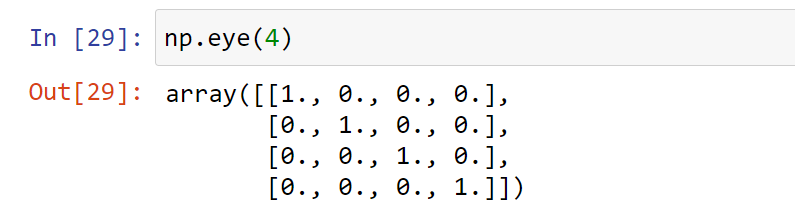### full((shape),value)

• Returns an array where all the elements will be the value you specified inside the full function

• Use a tuple inside the full function ,which defines the shape of an array

Example 22### arange(starting value,ending value,step size)

• Returns an 1-dimensional array where all the elements will be from starting value to the ending value (ending value will not be included)

• Same like range function in Python

Example 23### linspace(starting value,ending value,no of points)

• Returns an 1 dimensional array where the elements are an evenly or non-evenly spaced range of numbers from starting value to the ending value (ending value included)

Example 24### diag([values])

• Returns a diagonal array

• values should be passed inside a list or tuple format

Example 25## Extras:-

### Key difference between list and arrays

• Arrays can handle vectorized operation but list cannot

• That means, if you apply a function it is performed on every item in the array, rather than on the whole array object

Example 25• Once a NumPy array is created, you cannot increase its size, but we can do it on a list

Example 26• A NumPy array must have all items to be of the same data type, unlike lists. This is another significant difference

• List can hold any data types

Example 27### How to reverse the rows and the whole array?

• Reversing an array works like how you would do with lists, but you need to do for all the dimensions if you want a complete reversal.

Example 28

• Reverse only the row positions• Reverse only the column positions

Example 29• Reverse the row and column position

Example 30